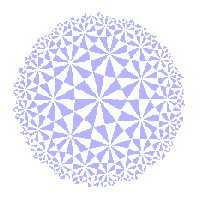# The Klein View of Geometry

The Hyperbolic Group

 We use the Poincare disc model of the hyperbolic plane. This has as its set of points the disc D = { z : |z| < 1 }. The disc has boundary the unit circle C = { z : |z| = 1}. The points of C do not belong to the geometry, but play a role similar to the "points at infinity" in euclidean geometry. We derive the hyperbolic group from a subgroup of the inversive group I(2), and develop the theory using ideas from inversive geometry. Let H(2) be the subgroup of I(2) consisting of those elements which map D to D and C to C. In fact it would be enough to demand that they map D to D, but this requires a continuity argument which would be out of place here. Since the elements of H(2) map D to D, we can consider their restrictions to D. This gives a group of transformations of D. Definition The hyperbolic group H(2) is the group consisting of the restrictions of the elements of H(2) to D. The elements are called hyperbolic transformations. Clearly H(2) is isomorphic to H(2). We shall usually ignore the distinction between an element of H(2) and its restriction to D. As inversive transformations, elements of H(2) may be direct (preserve the sense of angles) or indirect. Suppose that L is an extended line passing through O. Then inversion in L is essentially reflection. Since OεL, this maps C to C and D to D, so the inversion belongs to H(2). In particular, r0, reflection in the real axis, belongs to H(2). This maps z to z*, the complex conjugate. A knowledge of inversive geometry, and of inverse points in particular, is enough to establish our first major theorem. Theorem H1 If tεH(2) is direct, then t(z) = κ(z-c)/(c*z-1), If tεH(2) is indirect, then t(z) = (κ(z-c)/(c*z-1))*. where |c| < 1 and |κ| = 1 in each case. In the hyperbolic geometry pages, we observed that, if L is an i-line orthogonal to C, then iL, inversion with respect to L, maps C to C and D to D. Thus iLεH(2). Here, we establish a result stated on the hyperbolic geometry pages, namely that these maps (known as h-inversions) generate H(2). The proof requires the Origin Lemma from the hyperbolic geometry pages. Theorem H2 Any tεH(2) may be expressed as the composite of at most three h-inversions.